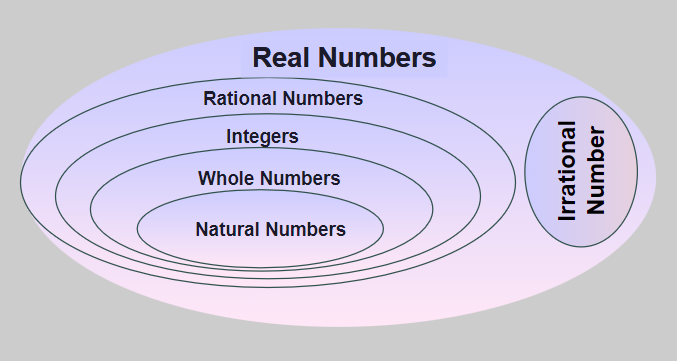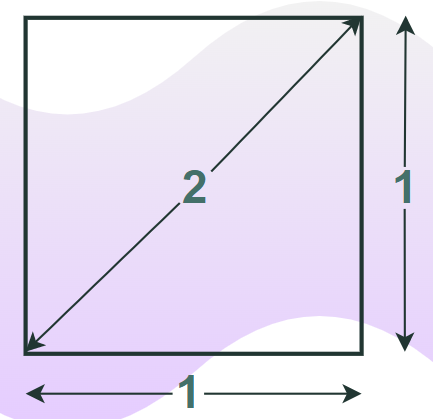Related Articles

# Irrational Numbers

Irrational Numbers are numbers that can not be expressed in the form of p/q where p and q are integers and q does not equal zero. Irrational numbers can not be represented via fractions or normal decimal values. They are non-recurring, non-terminating, and non-repeating decimals. They are part of real numbers but are different from rational numbers. We represent the Irrational number by the symbol Q’.

In this article, we will learn about irrational numbers, their properties, examples, identification, and others in detail.

## Irrational Number Definition

Irrational Numbers are numbers which can not be expressed as the ratio of two integers. They are a subset of Real Numbers and can be expressed on the number line. And, the decimal expansion of an irrational number is neither terminating nor repeating.

We can define irrational numbers as real numbers that cannot be expressed as p/q where p and q are integers and q ≠ 0.

## How to Identify an Irrational Number?

We know that irrational numbers are real numbers and they cannot be expressed in the form of p/q, where p and q are integers and q ≠ 0. For example, √ 5 and √ 3, etc. are irrational numbers. On the other hand, the numbers which can be represented in the form of p/q, such that, p and q are integers and q ≠ 0, are rational numbers.

## Examples of Irrational Numbers

√2, √3, π, e are some examples of irrational numbers.

• √2 = 1.41421356237309504880…
• Pi “π”= The value of π is 3.14159265358979323846264338327950… It is a really famous irrational number. People have calculated its value up to quadrillion decimals but still haven’t found any pattern yet.
• Euler’s number  “e” = Euler number is also very popular in mathematics. In this case, also, people tried calculating it up to a lot of decimals but still, no pattern was found. the value of e = 2.7182818284590452353602874713527 (and more …).
• Golden ratio  “ϕ” = This is an irrational number and its application is found in many fields like computer science, design, art, and architecture.

## Irrational Number Symbol

We represent the Irrational number with the symbol Q’ as Q represents the group of rational numbers so Q complement (Q’) is used to represent irrational numbers. Also,

Q U Q’ = R

where R is the set of real numbers.

## How to know a number is Irrational?

We know that rational numbers are expressed as, p/q, where p and q are integers and q ≠ 0. But we can not express the irrational number in a similar way. Irrational numbers are non-terminating and non-recurring decimal numbers. So if in a number the decimal value is never ending and never repeating then it is an irrational number.

Some examples of irrational numbers are,

• 1.112123123412345…
• -13.3221113333222221111111…, etc.

## Are Irrational Numbers Real Numbers?

Irrational numbers come under real numbers, i.e. all irrational numbers are real. But irrational numbers are different from rational numbers as they can’t be written in the form of fractions. Although, irrational numbers can be expressed in the form of non-terminating and non-recurring fractions. For example,  √2, √3, and π are all irrational numbers and can’t be written as fractions. The image below explains the relationship between Irrational numbers and Real Numbers.## Properties of Irrational Numbers

Various properties of irrational numbers are discussed below:

• Sum of two rational numbers may be rational or may be irrational.
• Sum of a rational number and an irrational number is an irrational number.
• Product of an irrational number with a non-zero rational number is an irrational number.
• Product of two irrational numbers may be rational or may be irrational.
• LCM of two irrational numbers may or may not exist.
• Set of irrational numbers is not closed under the multiplication process, but a set of rational numbers is closed.

## Product of Two Irrational Numbers

Product of two rational numbers may be either rational or irrational. For example,

• π × π = π2 is irrational.
• √2 × √2 = 2 is rational.

So Product of two Irrational Numbers can result in a Rational or Irrational Number accordingly

## Sum of Two Irrational Numbers

The Sum of two irrational numbers is sometimes rational sometimes irrational.

• 3√2 + 4√3 is irrational.
• (3√2 + 6) + (- 3√2) = 6, is rational.

### Product of Irrational Number and Non-zero Rational Number

The product of any irrational number with any non-zero rational number is an irrational number.

For example, 3×√2 is an irrational number as it can not be represented as p/q

### Fun Facts

Apparently, Hippasus (one of Pythagoras’ students) discovered irrational numbers when trying to write the square root of 2 as a fraction (using geometry, it is thought). Instead, he proved the square root of 2 could not be written as a fraction, so it is irrational. But followers of Pythagoras could not accept the existence of irrational numbers, and it is said that Hippasus was drowned at sea as a punishment from the Gods!

## Is Pi an Irrational Number?

Yes, Pi (π) is an irrational number because it is neither terminating decimals nor repeating decimals. We will learn more about Pi (π) as,

Let’s take a circle, measure its circumference, and divide it by its diameter. It will always be a constant if measured accurately.

This constant ratio is denoted by the Greek symbol π (read as pi). That is,

Circumference/diameter = π

It is an important universal constant, it occurs in lots of places in our universe and in our daily lives. It was not created by humans, it was discovered.

## What is the value of Pi

π = 3.14159265358979323846264338327950…

It is not infinite, it is an irrational number.

Note: We often take 22/7 as the value of Pi, but it is an approximation.

Now one might think, how is Pi irrational? One can measure the circumference, One can measure the diameter, and then take their ratio. So it must be rational as we define π as,

π = Circumference/Diameter

And if we define any number as that ratio of two numbers then it is a rational number. But while defining the ratio of π, we take approximation and the value of π is never exactly equal to the ratio of Circumference and Diameter.

### Is √2  an Irrational Number?

Yes, √2 is an irrational number because it is neither terminating decimals nor repeating decimals. But we can graphically represent the value of √2 as the diagonal of the square with sides one unit as shown in the image below.## List of Irrational Numbers

There are various irrational numbers that are widely used in mathematics. Some of the most commonly used irrational numbers are discussed in the table below,

## Irrational Number Theorem

The irrational number theorem is,

Theorem: If p is a prime number and p divides a2, then p also divides a.

Proof:

Using the Fundamental Theorem of Arithmetic

We can say that,

a = p1 × p2 × p3………..  × pn …..(i)

where,
p1, p2, p3, ……, pn are prime factors of a.

Squaring both sides of equation (ii)

a2 = (p1 × p2 × p3………..  × pn)2

a2 = (p1)2 × (p2)2 × (p3)2…….. × (pn)2

According to the Fundamental Theorem of Arithmetic. Every natural number has a unique prime factor.

Prime factors of a2 are p1, p2, p3……….., pn. Also, the prime factors of a are, p1, p2, p3……….., pn

Thus, if a2  is divisible by p, then p also divides a.

## How to Find Irrational Numbers?

An irrational Number between two prime numbers say a and b is given by √ab.

Irrational numbers between any two numbers are also found using the concept of perfect squares. We know that,

• √(1) = 1
• √(4) = 2

As the perfect squares of the number between them do not exist. So the numbers between them are irrational numbers, i.e. √(2), and √(3) are irrational numbers. Similarly the numbers

• √(4) = 2
• √(9) = 3

So the numbers between them, √(5), √(6), √(7), and √(8) are irrational numbers.

Also, the cube root of the non-perfect cube is an irrational number.

Read More, Irrational Number between 3 and 4

So we can say that the root of prime numbers is irrational numbers. √P  is an irrational number where P is the prime number.

This can be proved using the contradiction method.is

Statement: Square root of the prime number is an irrational number.

Proof:

Let us assume that, √u is a rational number.

By the definition of rational numbers

√u =p/q    …….(i)

Where p and q have no common factor other than 1 and q ≠ 0

Squaring both sides of equation (1), we have

u = p2/q2

p2 = uq 2    ………. (ii)

Now we can say that if u is a prime factor of p2, then u is a prime factor of p.

Thus,

p = u × c, where c is any integer.

from eq (ii)

(uc)2 = uq2

q2 = uc2…(iii)

From eq(iii) we can say that if u is a prime factor of q2, then u is a prime factor of q.

But initially we have assumes that p and q have no common factors. But from the above proof, we say that p and q have a common factor u, which implies that our initial assumption is wrong. That is √u is not a rational number. Thus, √u is an irrational number.

## Differences Between Rational and Irrational Numbers

The difference between rational and irrational numbers is discussed in the table below.

## Solved Examples on Irrational Numbers

Example 1: Find Rational Numbers or Irrational Numbers among the following.

2, 3, √3, √2, 1.33333…, 1.1121231234…

Solution:

• Rational Numbers: 2, 3, 1.3333…. are rational numbers
• Irrational Numbers: √3, √2, 1.1121231234… are irrational numbers

Example 2: Find the sum of the following irrational numbers.

a) √2, √2  b) √2, √3

Solution:

a) √2 + √2 = 2√ 2 (they are added as two like variables)

b) √2 + √3 = √2 + √3 (they can’t be added as unlike variables)

Example 3: Find the product of the following rational numbers.

a) √2, √2  b) √2, √3

Solution:

a) √2 × √2 = 2

b) √2 × √3 = √6

## FAQs on Irrational Numbers

### Q1: What is the Irrational Number definition?

Irrational numbers are the numbers that are not defined as p/q where p and q are integers with no common factors and q does not equal to zero. They are non-terminating and non-recurring decimal numbers whose exact value can never be found.

### Q2: What are Examples of Irrational numbers?

The examples of rational number are,

• √2 = 1.414…
• √3 = 1.732…
• Pi (π) = 3.1415…

### Q3: Are Integers Irrational Numbers?

No, Integers are not irrational numbers but are rational numbers as they can easily be represented in, p/q form. For example integers like, -1 and 2 are represented in rational number form as,

• -1 = -1/1
• 2 = 2/1

### Q4: How can you Identify an Irrational Number?

Irrational number are the numbers which are non-recurring and non-terminating decimals and we can easily identify them using this property. For example,

• 1.123123123…. is a rational number as it is a recurring decimal.
• 1.123123 is a rational number as it is a terminating decimal.
• 1.1121231234…. is an irrational number as it is non-recurring and non-terminating decimal.

### Q5: Why Pi is an Irrational Number?

Pi(π) is an irrational number because it can not be expressed in the form of p/q and it is a non-recurring and non-terminating decimal and its value is,

π = 3.14156162…